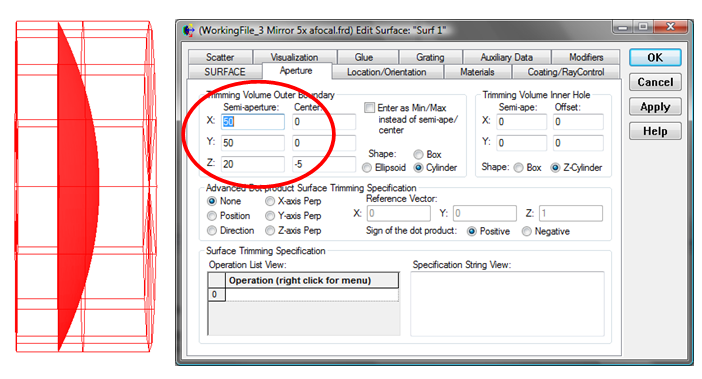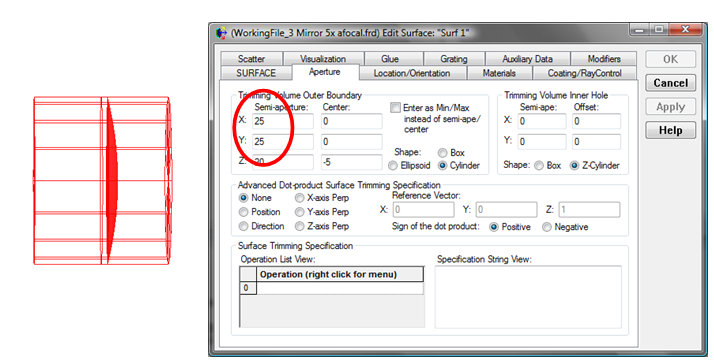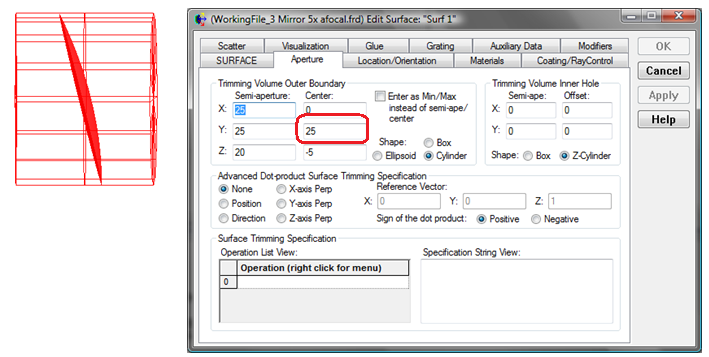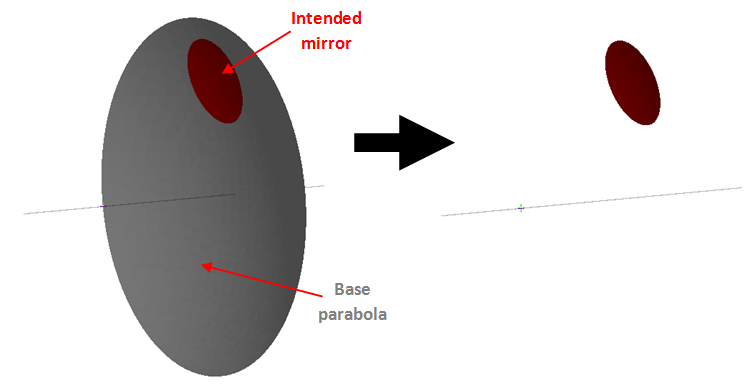# Defining Off-Axis Parabolas

This article discusses how to define the off-axis parabolas (“OAPs”) using apertures to select the off-axis portion of the base parabola.

When a surface is defined to be parabolic, the shape of the parabola is defined via a radius and a conic constant (“kappa”). However, this does not define the size of the surface; this is defined by the surface “aperture.” This is demonstrated in the figure below where the parabola radius and kappa are the same in both cases, but with a smaller x,y aperture in the second case.The red wire frame seen in the Visualization View denotes the aperture volume. You can see that in this case the parabolic surface is bound by the x and y size of the aperture. Moving the aperture “Center” away from the parabola axis allows us to select a region of the parabola that is “off axis”. This is demonstrated in the figure below which uses an aperture size that is the same as previous but with the vertical center shifted “off axis” by 25 units.It is important to note that this surface does not have the same shape as the previous one (i.e., it is not just moved and re-orientated). It is defined by the solution to the parabola equation centered around (x,y) = (0,25) in the bounds specified by the Semi-aperture values.

So, in summary when defining an optical system that uses OAPs, the engineer needs to first define the base parabola, and then set the aperture to define the piece that becomes the mirror.